# Rotation Curve of Nearby Galaxies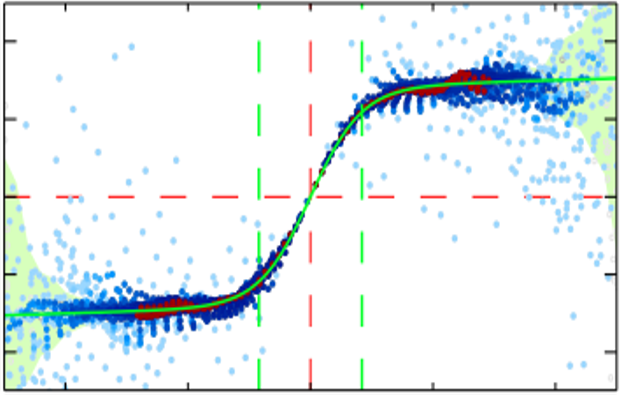Abstract: We measure rotation curves of SDSS-IV MaNGA galaxies and study their dependence on mass and environmental parameters. We perform PSF deconvolution on the 3D Cube to overcome the atmospheric seeing and the beam-smearing effect. Using 2D stellar velocity maps measured from the deconvolved cubes, we fit a simple thin-disk rotation curve model (a hyperbolic tangent function with a linear term) to obtain model parameters and kinematic geometry of the galaxies. The PSF deconvolution is crucial to study the detail of the rotation curve. We notice that galaxies with disk show clear rigid body rotation around the center and flat rotation at their outskirts, but also show inclined rotation curve (decreasing/increasing) at their outskirts as well, depending on galaxy morphology (Early/Late). Rotation curve model parameters (rotation velocity amplitude, inner specific radius, and outskirt curve slope) are correlated with mass, for both early and late-type galaxies. For low mass (log(M_star/M_sun) < 10) late-type galaxies, their rotation curve slope at the outskirt have a positive correlation when the large-scale (background mass density from 20 nearest galaxies) or the small scale (projected distance to the nearest neighbor galaxies) environmental parameters are larger than the mean cosmic mass density or smaller than one virial radius of the nearest neighbor galaxy. These results suggest that both the large and small scale environmental parameters play a considerable effect on stellar kinematics.

## Data

We use SDSS-IV MaNGA MPL-6 data (Bundy et al. 2015) (equivalent to DR15), which includes 3D cube data from 4,601 galaxies.. We utilize Sersic index, ellipticity, and elliptical Petrosian radius, redshift, and stellar mass from NASA Sloan Atlas as well as morphology infromation of 4,419 galaxies from KIAS value-added galaxy catalog (Choi et al. 2010).

## Methods

• PSF Deconvolution & Velocity Measurement
We perform PSF deconvolution on all MaNGA MPL-6 data, and measure line of sight velocity distribution by pPXF, using Miles stellar population synthesis models. We fit the 2D rotation curve model (hyperbolic tangent + linear function) on the 2D obtained velocity maps and obtain model parameters as below.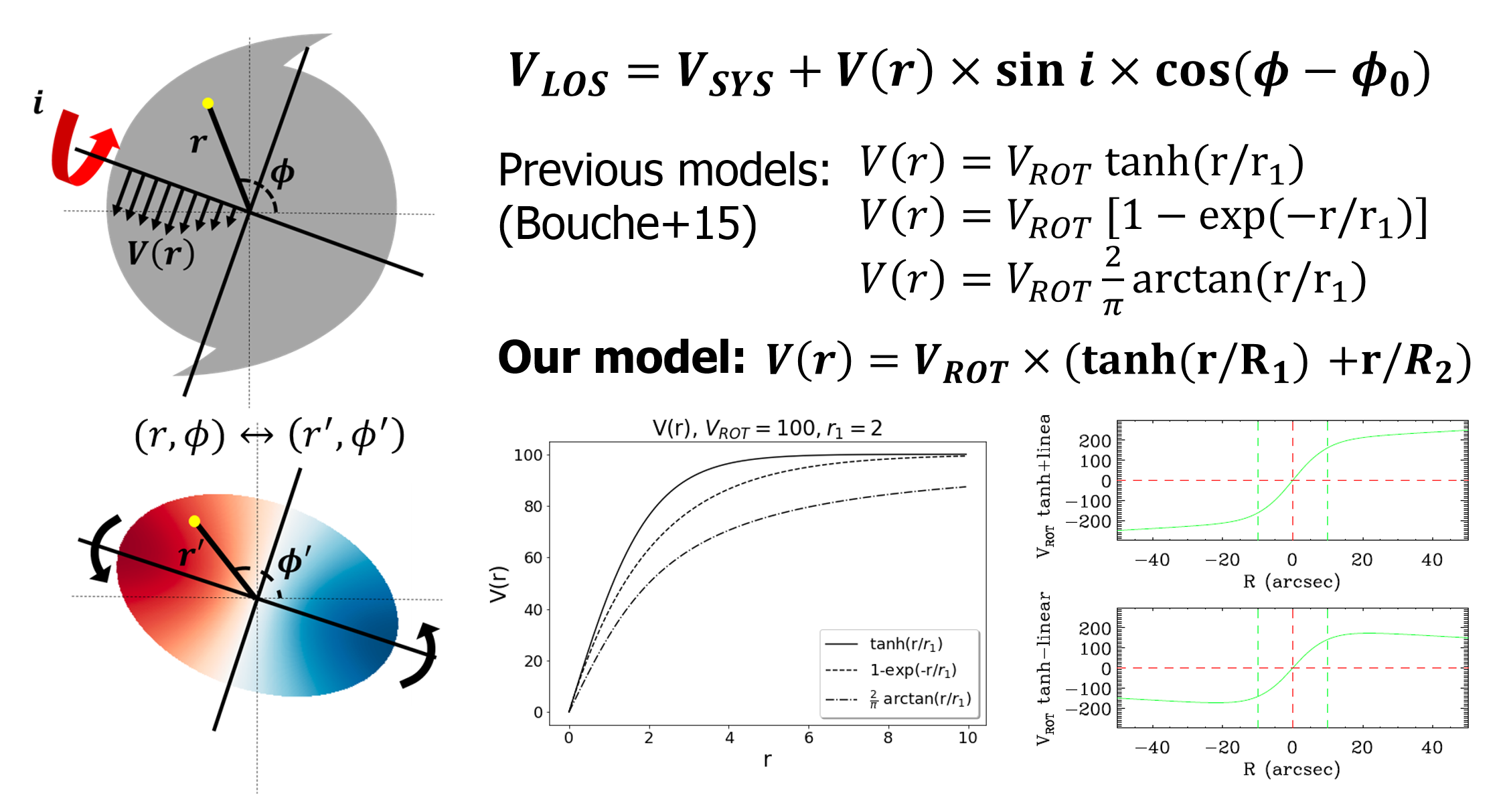Rotation of the disk galaxy can be captured only when they are inclined. Previously, its 2D velocity distribution shape was described by three functions which are mentioned in the figure. By adding one linear term on the previous model, we can fit the slope of the galaxy rotation curve at its outskirts.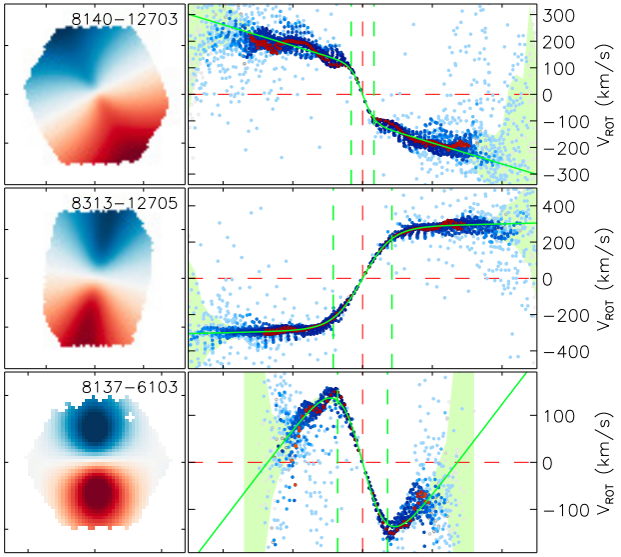Here we show examples of our 2D velocity model fitting. (left) 2D model velocity map which from best-fit 2D rotation curve model. (right) The rotation curve of example MaNGA galaxies. The green line indicates rotation curve from the fitted model.
• Large-scale environmental parameter, $\rho_{20}$ (Park et al. 2008)
Background mass density using the (3D) closest 20 galaxies (several Mpc scale), normalized by mean mass density

• Small-scale environmental parameter, $R_{n}$ (Park et al. 2009)
Distance to the nearest neighbor in a unit of the neighbor’s virial radius

## Results

Below results are from MaNGA late-type galaxies with $R_{major}$/$R_1$ > 3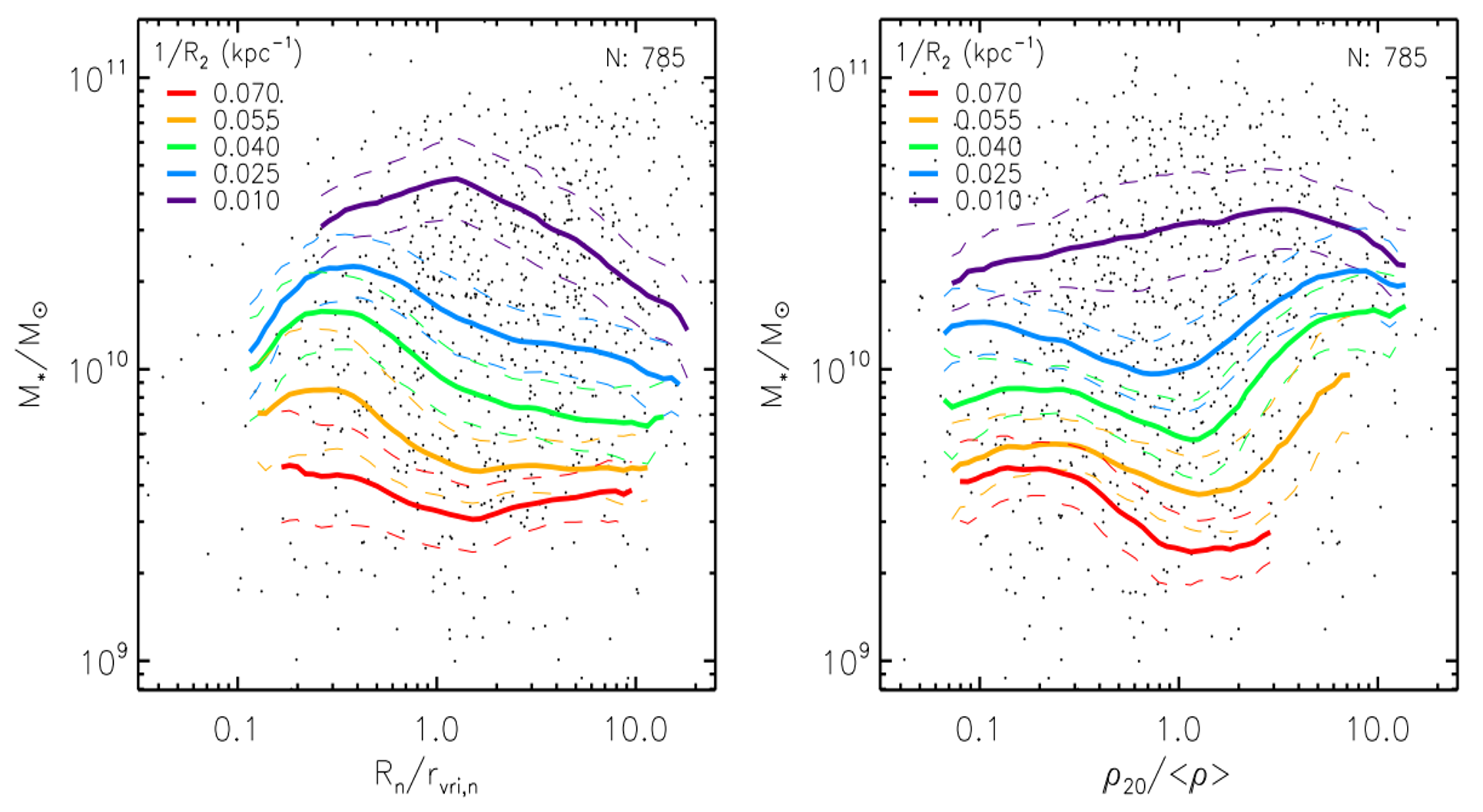↑ $1/R_2$ over small/large scale environ. parameter versus galaxy mass (left/right). There is clear $1/R_2$ dependence on galaxy mass, as well as a weak correlation with large/small scale environmental parameters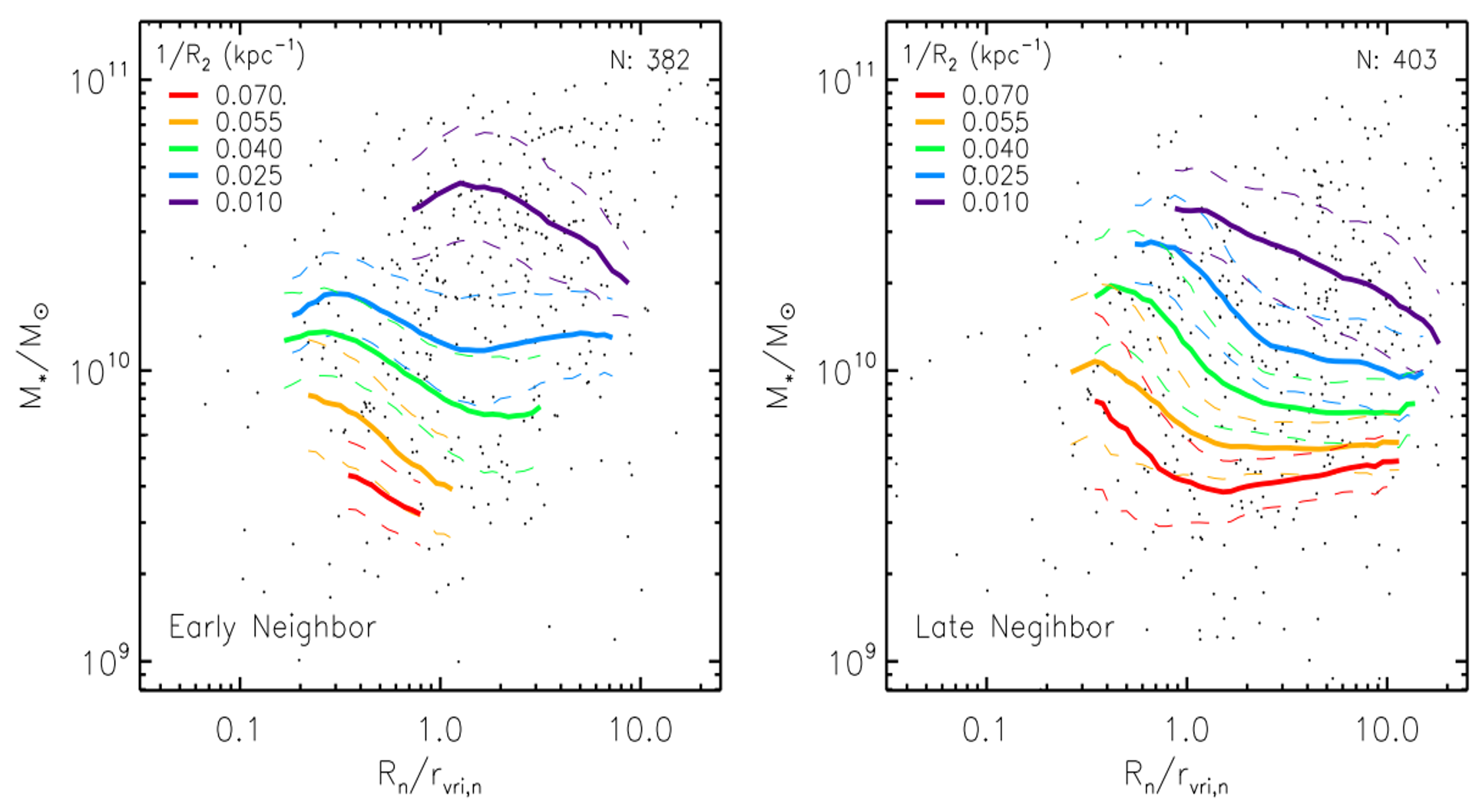↑ $1/R_2$ over small scale environ. parameter versus galaxy mass, depending on neighbor galaxy morphology. Left: early neighbor, right: late neighbor. There is steeper change on $1/R_2$ around $R_n/r_n=1$ when the neighbor is late type.

## Summary

• We deconvolved MaNGA 3D cube data to mitigate the effect of large PSF size.
• The deconvolution procedure is crucial to recovering true RC model parameters.
• MaNGA rotation curve is parametrized by a fitting model function.
• Rotation curve slope at galaxy outskirts radius shows correlation with large/small scale environment parameter at fixed stellar mass, especially when R_n/r_vri,n<1 and $\rho$_20/<ρ> >1.##### Haeun Chung
###### Assistant Research Professor / Aspera Project Scientist

An astronomer who builds telescopes and instruments, studies circuumgalactic medium and kinematics of galaxies.